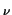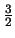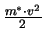Next: 3.1 Sets of Partial Up: Dissertation Grasser Previous: 2.3.3.14 Distributed Device

# 3. Device Equations

In MINIMOS-NT carrier transport can be treated by the drift-diffusion (DD) and the hydrodynamic (HD) transport models. For either carrier type the transport model can be chosen independently. In addition, the lattice temperature can be treated either as a constant or as an unknown governed by the lattice heat flow equation. These equations will be reviewed in Section 3.1. It is worth mentioning that there is some confusion in the literature about the HD transport model. When deriving the HD model from Boltzmann's transport equation, the average carrier energies wread

 w=. kB . T+(3.1)

with v being the average carrier velocity. For the derivation of (3.1) a momentum displaced Maxwellian has been assumed [26,3]. When the second term in (3.1) and other related terms in the HD equations are neglected, the energy-transport model is obtained . Depending on these simplifying assumptions, different energy-transport models have been used in device simulators. As it is common practice to refer to the simplified energy-transport model as hydrodynamic transport model, the same nomenclature is used in MINIMOS-NT and in this thesis.

The resulting equation system for the most general case is very complex and time consuming to solve. Simplifications should be made whenever possible, e.g., DD model instead of HD model or to completely neglect carrier transport by assuming a constant quasi-Fermi level for the respective carrier type. However, the validity of these simplifications must be carefully investigated. This is normally done by comparison of simulation results for different equation sets. Despite the obvious fact that depending on the equation set different principal physical effects are taken into account, e.g., self- and carrier-heating, the influence on the models for the physical parameters is more subtle. The main reason for this is that in the case of the HD model, information about the average carrier energy is available in form of the carrier temperature. Many physical parameters depend on this average carrier energy, e.g., the mobilities and the energy relaxation times. In the case of the DD model the carrier temperatures are assumed to be in equilibrium with the lattice temperature, that is TC = TL, hence, all energy dependent parameters have to be modeled in a different way. The carrier energies are estimated using the local energy balance equations which give expressions for the carrier temperatures as a function of the local electric field. These expressions, however, are only valid under homogeneous conditions. Models for the physical parameters for the DD and HD case will be called consistent when they deliver equivalent results under these homogeneous conditions as will be shown.Next: 3.1 Sets of Partial Up: Dissertation Grasser Previous: 2.3.3.14 Distributed Device
Tibor Grasser
1999-05-31